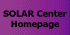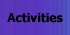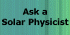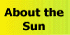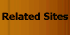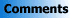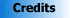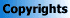How Galileo Used Mathematics to Prove the Spots Were on the Sun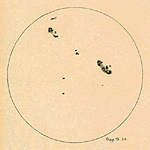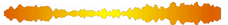Let's describe a sunspot's movement geometrically, as Galileo did: Look at the figure, where the circle represents a view of the Sun from above and "you" represents you, an observer on Earth.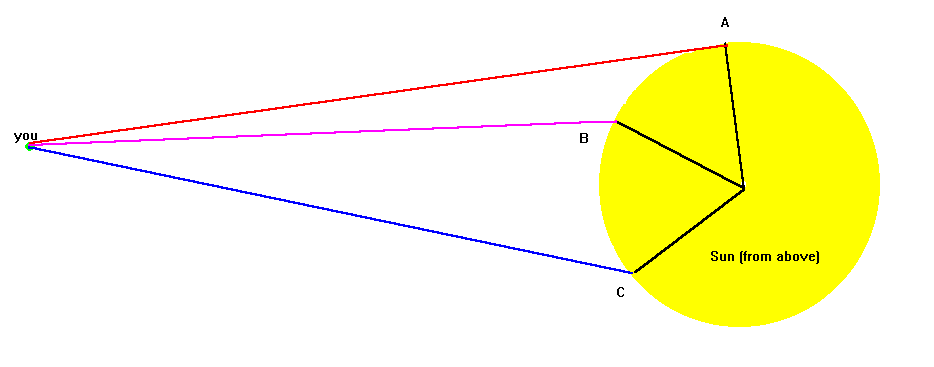The points A, B, and C are points taken at equal distances on the surface of the Sun, and thus represent positions of a sunspot at equal intervals of time. The apparent motion from A to B, as seen by you, is measured by the angle A-you-B. Compare it to the angle B-you-C, which is obviously much larger. Since the time and distance are the same for A-you-B and B-you-C, the spot appears to move much more slowly as it rounds the edge of the Sun than when it passes across the middle of the disk.On the other hand, if the spot were a planet revolving around the Sun, as shown by the black circle, the angles A-you-B and B-you-C differ by only a very small amount.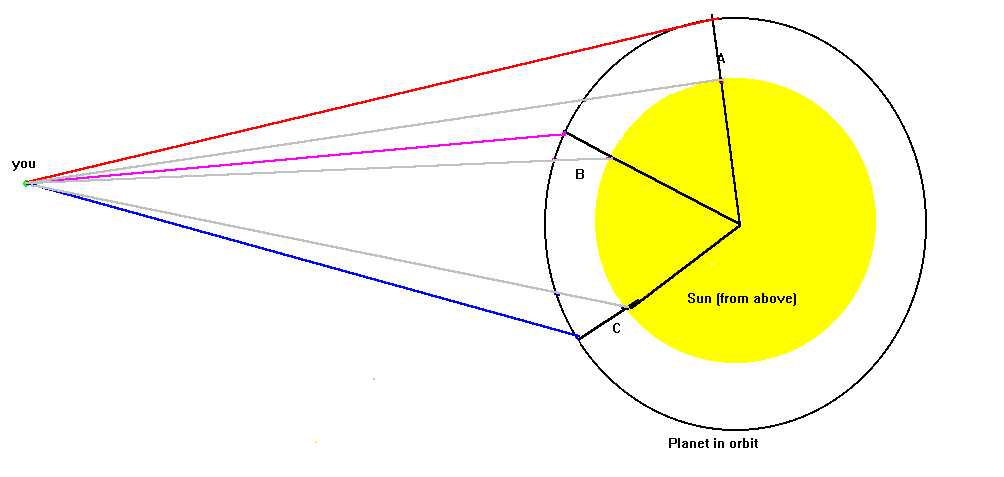```

``````
```

##Now, can you do a calculation to express these results mathematically?

```

``````
```

##Back To "Galileo's Proof"

```

``````

```